# Working with Excel Files in Julia

Julia is a high-level open-source programming language meaning that its source is freely available. It is a language that is used to perform operations in scientific computing. Julia is used for statistical computations and data analysis. Julia provides its users with some pre-defined functions and built-in packages with the help of which Julia makes it possible to work with Excel Files, that too with quite an easiness.

With the help of Packages, Julia makes is easier to Read an Excel File. First, the package that is needed to be added to get its functionality for reading the Excel file is

```XLSX.jl package
```

All the packages can be added with passing it as an argument in the add(” “) function which is present in the Pkg object

```Pkg.add("")
```

#### Reading one row at a time

To read one row at a time from an Excel File, Julia provides a function eachrow() to iterate over each row of the file and store the same in a variable.

Approach:

• First, add the package XLSX
• Open the file using openxlsx() function with arguments passed in strings as the name of the file, accessing the cached memory.
• Now we will traverse the sheet in which our data is stored with the help of a loop and a variable to store(sheet) while passing.
• Now to access each row of the table we need to iterate row by row with help of function named eachrow() passing sheet as an argument in it.
• The variable to iterate used by for loop is known as ‘SheetRow‘ values are read using column references.
• row_number(iterating_variable) function is used to access the row number and the iterating variable is passed in it which will help in traversing.
• Now to read the referenced column the variable passed should have its argument having the same type as the values contained in the particular header and stored in another variable. r[“B”] for string,r for int values etc.
• Now just print the variable in which these data are stored and ending both the loops

## Julia

 `using Pkg ` `Pkg.add(``"XLSX"``) ` `XLSX.openxlsx(``"sample1.xlsx"``, enable_cache``=``false) do f ` `  ``sheet ``=` `f[``"Sheet1"``] ` `  ``for` `r ``in` `XLSX.eachrow(sheet) ` ` `  `    ``# r is a `SheetRow`, values are read  ` `    ``# using column references ` `    ``rn ``=` `XLSX.row_number(r) ``# `SheetRow` row number ` `    ``v1 ``=` `r[``1``]    ``# will read value at column 1 ` `    ``v2 ``=` `r[``2``]``# will read value at column 2 ` `    ``v3 ``=` `r[``"B"``] ` `    ``v4 ``=` `r[``3``] ` `    ``println(``"v1=\$v1, v2=\$v2, v3=\$v3, v4=\$v4"``) ` `  ``end ` `end`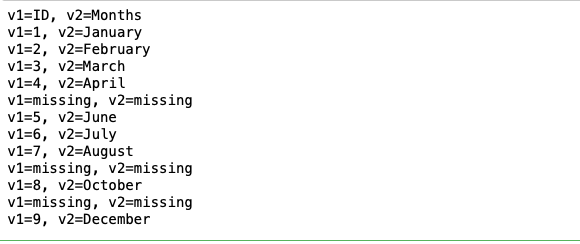#### Reading whole file at once

Julia provides a function readxlsx() to read all the contents of a file in a single attempt.

Approach:

• First, add the package Pkg
• Then add the XLSX package using add function and passing the package name as the argument
• The file can be read with the output of its all information by a  function readxlsx() which reads the xlsx files
• Now this function returns the dimension of all the sheets inside the xlsx file.
• These dimension of all the sheets can be stored in a separate variable and then it can be accessed by passing a string.
• Now the separate variable’s all data in this sheet passed can be viewed with double colon which gives access to read all the data inside this particular sheet.

## Julia

 `using Pkg ` `Pkg.add(``"XLSX"``) ` `import` `XLSX ` `xf ``=` `XLSX.readxlsx(``"sample3.xlsx"``)`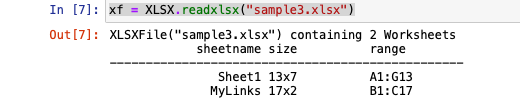## Julia

 `sh ``=` `xf[``"Sheet1"``] ` `sh[:]`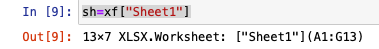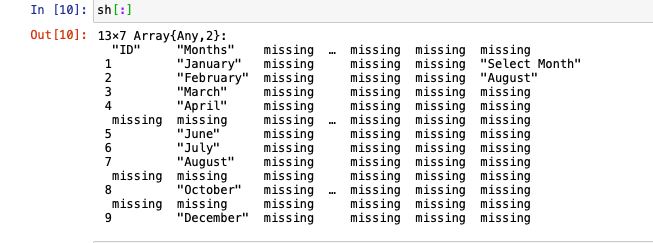#### Modifying contents of a File

Modification of contents of an Excel File can be done by opening the file in the ‘rw’ i.e. Read-write mode and then further updating the values with the help of iterator.

Approach:

• First, add the package Pkg
• Now open the file in the ‘rw‘ mode to make changes in the existing file.
• Now traverse with sheet variable with help of a loop
• Now pass the row number into the sheet variable to access the row
• Replace it with the new number or string
• END the loop using ‘end’.

## Julia

 `# Modifying contents of a file ` `using Pkg ` `Pkg.add(``"XLSX"``) ` `XLSX.openxlsx(``"sample3.xlsx"``, mode``=``"rw"``) do xf ` `    ``sheet ``=` `xf[``1``] ` `    ``sheet[``"B2"``] ``=` `"March"` `#row number = B2 ` `end`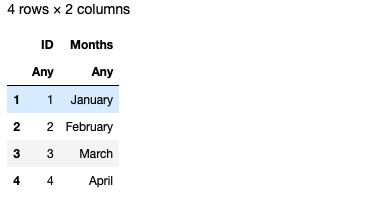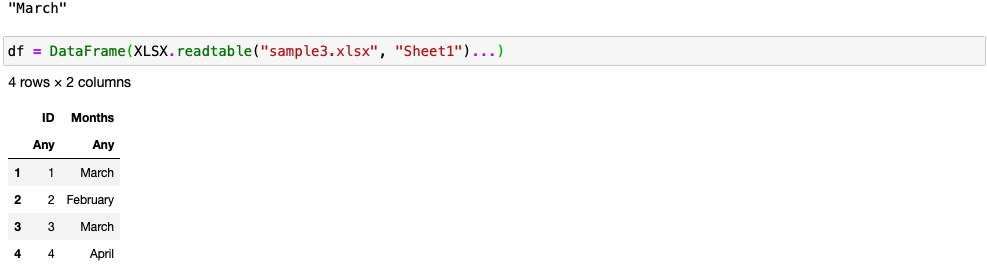#### Creating new columns

The addition of columns in an Excel File is done by opening the file in ‘rw’ mode and then using the collect() function.

Approach:

• First, add the packages Pkg, XLSX, and DataFrames.
• Now open the file in the ‘rw‘ mode means editing an existing file
• Now traverse the sheet using variable
• Pass the arguments as the column number in which you want to add the column and the dimension
• And pass the function collect passing arguments in it telling the range
• Then end the loop

## Julia

 `# Only 10 columns are present ` `df2 ``=` `DataFrame(XLSX.readtable(``"sample2.xlsx"``, ``"Sheet1"``)...) ` ` `  `# add a new column to an existing file  ` `# and makes it 11 columns ` `XLSX.openxlsx(``"sample2.xlsx"``, mode``=``"rw"``) do xf ` `    ``sheet ``=` `xf[``1``] ` `     `  `    ``# add a column from "K1" to "K3" ` `    ``sheet[``"K1"``, dim``=``1``] ``=` `collect(``1``:``3``) ` `end`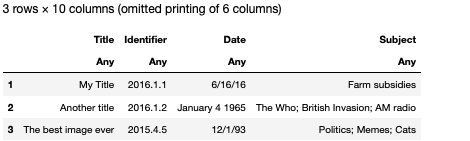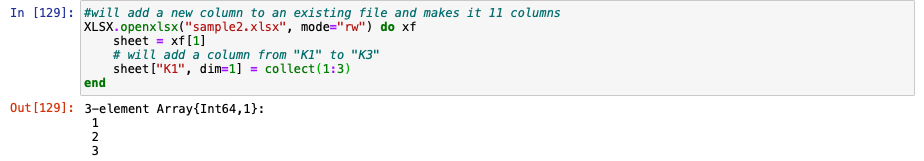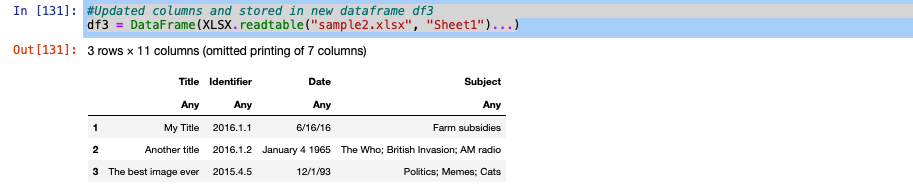#### Deleting contents of a file

The deletion of contents from an Excel File can be done by using the setdiff() function. This function compares all the rows and removes the row that is passed as an argument.

Approach:

• First tell the row that is needed to be deleted.
• Now in the same DataFrames ‘df’ pass the arguments using setdiff() function in which it reads all the rows from starting and deletes the row passed.

## Julia

 `# deleting from an existing column ` `row ``=` `2` `df ``=` `df[setdiff(``1``:end, row), :]`

Original File: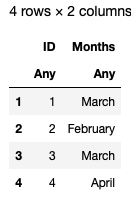Updated File: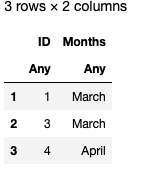#### Appending to files

Julia provides a function append() to perform the append operation to files. This function takes both the dataframes as an argument and returns the appended dataframe.

Approach:

• To append the file use the append() function passing the DataFrames (df2,df3) as arguments
• The append() function works as adding the df2 dataframe at the back of df3 dataframe
• But remember the columns should be the same in both the dataframes (df2,df3)

## Julia

 `# Only 10 columns are present ` `df2 ``=` `DataFrame(XLSX.readtable(``"sample2.xlsx"``, ``"Sheet1"``)...) ` ` `  `# Add a new column to an existing file  ` `# and makes it 11 columns ` `XLSX.openxlsx(``"sample2.xlsx"``, mode``=``"rw"``) do xf ` `    ``sheet ``=` `xf[``1``] ` `     `  `    ``# will add a column from "K1" to "K3" ` `    ``sheet[``"K1"``, dim``=``1``] ``=` `collect(``1``:``3``) ` `end ` ` `  `# Updated columns and stored in new dataframe df3 ` `df3 ``=` `DataFrame(XLSX.readtable(``"sample2.xlsx"``, ``"Sheet1"``)...) ` ` `  `# Appended df2 rows to the end of df3  ` `# with same column names ` `df3 ``=` `append!(df2,df3)`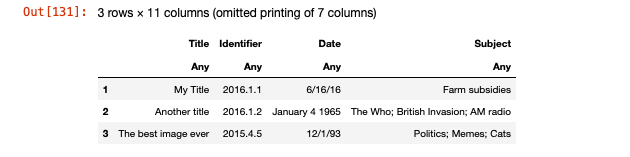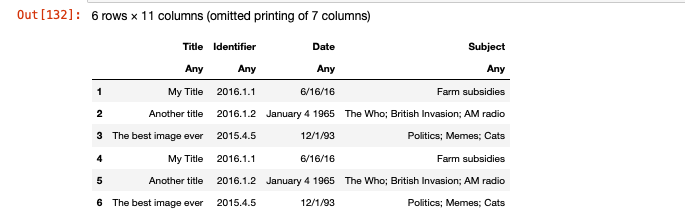#### Writing a new excel file

To write contents to a new Excel file, open the file in the ‘w’ i.e. write mode and then use the collect() function to add columns to the files and further assign the values to be added to the variables.

Approach:

• First, open the file in the writing mode ‘w’ and start the loop
• Now to add a row to the new file give the row number as an argument in the sheet variable and pass the collect function giving range till which we want a row and pass them as arguments
• Now to add the column to the file pass the row number and dimension as 1 for column
• Pass the collect() function into the sheet variable and pass range in the collect() function till which we want our column, on the particular row number
• Now pass a matrix of numbers in the sheet variable separated by semicolon(;)  in square brackets and pass the row numbers range in the sheet variable till which we want our matrix.

## Julia

 `# Writing a new xlsx file and  ` `# the mode is w means(write) ` `# and created a new one. ` `XLSX.openxlsx(``"sample5.xlsx"``, mode``=``"w"``) do xf ` `    ``sheet ``=` `xf[``1``] ` `     `  `    ``# add a row from "A5" to "E5" ` `    ``# equivalent to `sheet["A5", dim=2] = collect(1:4)` ` `    ``sheet[``"A5"``] ``=` `collect(``1``:``5``) ` ` `  `    ``# will add a column from "B1" to "B4" ` `    ``sheet[``"B1"``, dim``=``1``] ``=` `collect(``1``:``3``) ` ` `  `    ``# will add a matrix from "A7" to "C9" ` `    ``sheet[``"A7:C9"``] ``=` `[ ``1` `2` `3` `; ``4` `5` `6` `; ``7` `8` `9` `] ` `end `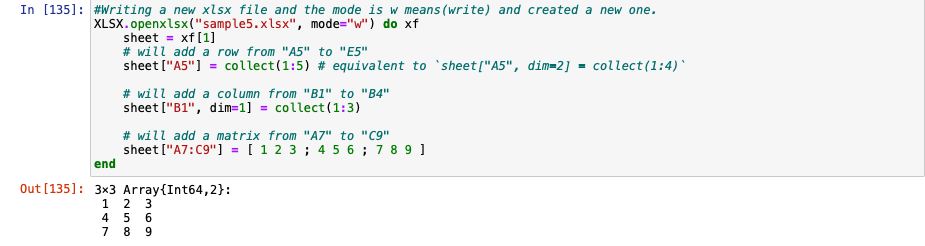My Personal Notes arrow_drop_upCheck out this Author's contributed articles.

If you like GeeksforGeeks and would like to contribute, you can also write an article using contribute.geeksforgeeks.org or mail your article to contribute@geeksforgeeks.org. See your article appearing on the GeeksforGeeks main page and help other Geeks.

Please Improve this article if you find anything incorrect by clicking on the "Improve Article" button below.

Article Tags :

Be the First to upvote.

Please write to us at contribute@geeksforgeeks.org to report any issue with the above content.